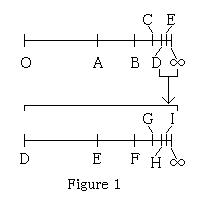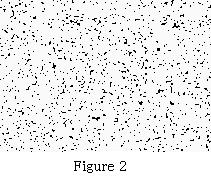﻿
```April 14, 2016
A new cosmology based on observation results and some hypotheses  Japanese
Y. Funatsu
Mathematician, Statistician, Doctor of science

Summary: Our cosmos is infinite. There is no expansion.
・ Observation result: Celestial bodies far from the earth are receding.
・ Hypothesis a: Inside of our cosmos is uniform from a broader standpoint.
・ Hypothesis b: Nothing is faster than light.

Ⅰ　Size of our cosmos is infinite.
1. It is said that our cosmos was small in its early time. It is also said that our cosmos grew bigger by expansion which
continues still now. The size of our cosmos is considered finite there. This clearly means that there is another space
surrounding our cosmos. Another space can be said outer (or parent) cosmos of ours. Hence our cosmos is just a celestial
body in the outer cosmos. This is what is derived from present cosmology.
On the other hand, Funatsu insists that the size of our cosmos is infinite when measuring from inside, but is finite
when measuring from outer cosmos. It means that there are two standards to measure our cosmos.

2. Funatsu develops a logic based on above mentioned three. It is a special importance that we are measuring cosmos by
means of light. From hypothesis a, the size of our cosmos must be infinite. The reason is as follows.
Celestial body(1) has been observed 130×108 light-years(ly) distant from the earth. From hypothesis a, celestial body(2)
should be observed 130×108 ly distant from celestial body(1). Hence we know that celestial body(2) is 260×108 ly
distant from the earth. Moreover, celestial body(3) should be observed 130×108 ly distant from celestial body(2).
Hence we know that celestial body(3) is 390×108 ly distant from the earth. Repeating this, celestial body must exist in
infinite distance. Hence our cosmos is infinitely big. This measurement is from the earth, that is, from inside of our
cosmos. If our cosmos is finite by the observation from inside, hypothesis a must be rejected.

3. Cosmos has a border. From hypothesis b, we can define that the distance between two spots is equivalent to time in which
light runs between the two. From section 2, light that started from a spot takes infinite time to arrive at the border of
the cosmos. Hence the border of cosmos is infinitely distant. This means that the size of our cosmos is infinite.
However, our cosmos is finite when observed from outer cosmos, because our cosmos is a celestial body in outer cosmos.

4. We have two standards of measurement about cosmos, that is, standard of inside and standard of outside. Figure 1 showsthe inside of our cosmos. O denotes a spot in our cosmos. ∞ denotes the border of our cosmos,
that is, the destination of the running light. A, B, C, D, E,... are the spots which the light
passes. Light goes from O to A→B→C→D→E→........→∞. The time that the light runs intervals
O－A, A－B, B－C, C－D, D－E,......are equal. However, the intervals are not equal in figure 1.
The farther from O, the narrower the intervals. Intervals near the destination are infinitesimal.
This is for the sake of figure’s convenience. Enlarging D－∞, D,E,F,... are also spots which
the light passes. The light takes equal time to pass each interval. Therefore the light takes
infinite time to arrive at the border of cosmos. Hence our cosmos is infinite.

Ⅱ　Cosmos neither grows nor becomes small. There is no expansion.
5. If we assume our cosmos is finite, the observation result means that our cosmos is growing.
But if our cosmos is infinite, the size of our cosmos does not vary, even if the observation
result is true.

6. If we assume our cosmos is finite, we can say that our cosmos is expanding and was small at the early time. However,
if our cosmos is infinite, it was infinite at the early time too, and there is no relationship between expansion and
the observation result.

Ⅲ　Cosmos shuts light up.
7. Light that started from a spot in cosmos can not go to outside of the cosmos. The power that shuts light up into the
cosmos is strong gravity toward inside. Matters have two powers i.e. powers of dispersion and gathering. The movement
that celestial bodies are receding is against the gravity. These two powers maintain the balance of cosmos.

Ⅳ　The ratio of any finite part of cosmos to whole cosmos is infinitesimal.
8. Any finite part of our cosmos, even if it is enormously big, is infinitesimal in the ratio to whole cosmos.

Ⅴ　Phenomena occur with probability.
9. Phenomena in cosmos occur by equal probability because of hypothesis a. Equal probability causes variance.An example: If we expose a grid pattern short time in rain, the number of raindrops in each
grid will not be equal, but, for example, 1, 2, 4, 0,.... We have a variance of these numbers.
Figure 2 shows a distribution of dots which consist of sets of two random numbers. Random
numbers occur with equal probability. The appearance is not homogeneous but we can see
variation of the density of  dots. Distribution of matters and energy in the cosmos are not
homogeneous but uniform with the state that has variation. The variations form hierarchy.
Matters and energy are floating in space. The state varies with time. Thus our cosmos is
uniform from a broader standpoint.

Ⅵ　Other items(Omitted)
10. Birth and death of cosmos. Big explosion. Geocentric theory and heliocentric theory in cosmos.
Nature of infinity in mathematics. How to count the number of celestial bodies, etc.

---------------------------------------------------------------------------------
(Contact)
Y. Funatsu
Hanakoganei 2-6-1, Kodaira-shi, Tokyo, 187-0002, Japan
Email  funatsu@mvf.biglobe.ne.jp

```